Updating search results...

# 7 Results

View
Selected filters:
• square-roots-and-pythagorean-theorem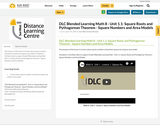Conditional Remix & Share Permitted
CC BY-NC-SA
Rating
0.0 stars

The purpose of this lesson is to learn about square numbers and perfect squares by using an area model.

Included is a YouTube video to support Grade 8 Blended Learning Math - Unit 1.1: Square Roots and Pythagorean Theorem - Square Numbers and Area Models.

Subject:
Math
Material Type:
Activity/Lab
Homework/Assignment
Lesson
Provider:
Sun West Distance Learning Centre (DLC)
06/14/2019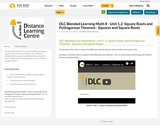Conditional Remix & Share Permitted
CC BY-NC-SA
Rating
0.0 stars

The purpose of this lesson is to learn the difference between squares, square numbers and square roots.

Included is a YouTube video to support Grade 8 Blended Learning Math - Unit 1.2: Square Roots and Pythagorean Theorem - Squares and Square Roots.

Subject:
Math
Material Type:
Activity/Lab
Homework/Assignment
Lesson
Provider:
Sun West Distance Learning Centre (DLC)
06/14/2019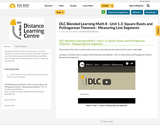Conditional Remix & Share Permitted
CC BY-NC-SA
Rating
0.0 stars

The purpose of this lesson is to learn about we can use the properties of a square to find its area or side length.

Included is a YouTube video to support Grade 8 Blended Learning Math - Unit 1.3: Square Roots and Pythagorean Theorem - Measuring Line Segments.

Subject:
Math
Material Type:
Activity/Lab
Homework/Assignment
Lesson
Provider:
Sun West Distance Learning Centre (DLC)
06/14/2019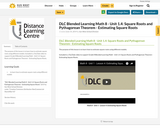Conditional Remix & Share Permitted
CC BY-NC-SA
Rating
0.0 stars

The purpose of this lesson is to learn how to estimate square roots using different models.

Included is a YouTube video to support Grade 8 Blended Learning Math - Unit 1.4: Square Roots and Pythagorean Theorem - Estimating Square Roots.

Subject:
Math
Material Type:
Activity/Lab
Homework/Assignment
Lesson
Provider:
Sun West Distance Learning Centre (DLC)
06/14/2019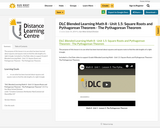Conditional Remix & Share Permitted
CC BY-NC-SA
Rating
0.0 stars

The purpose of this lesson is to use what has been learned about squares and square roots to find the side lengths of a right triangle.

Included is a YouTube video to support Grade 8 Blended Learning Math - Unit 1.5: Square Roots and Pythagorean Theorem - The Pythagorean Theorem.

Subject:
Math
Material Type:
Activity/Lab
Homework/Assignment
Lesson
Provider:
Sun West Distance Learning Centre (DLC)
06/14/2019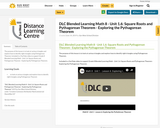Conditional Remix & Share Permitted
CC BY-NC-SA
Rating
0.0 stars

The purpose of this lesson is to look at various triangles and explore how to identify right triangles using Pythagorean Theorem.

Included is a YouTube video to support Grade 8 Blended Learning Math - Unit 1.6: Square Roots and Pythagorean Theorem - Exploring the Pythagorean Theorem.

Subject:
Math
Material Type:
Activity/Lab
Homework/Assignment
Lesson
Provider:
Sun West Distance Learning Centre (DLC)
06/14/2019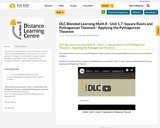Conditional Remix & Share Permitted
CC BY-NC-SA
Rating
0.0 stars

The purpose of this lesson is to use the Pythagorean Theorem and its corresponding algebraic expression solve a variety of problems.

Included is a YouTube video to support Grade 8 Blended Learning Math - Unit 1.7: Square Roots and Pythagorean Theorem - Applying the Pythagorean Theorem.

Subject:
Math
Material Type:
Activity/Lab
Homework/Assignment
Lesson
Provider:
Sun West Distance Learning Centre (DLC)